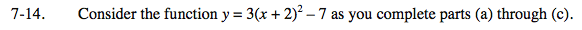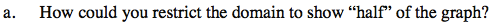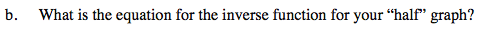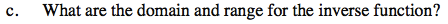Home > INT3 > Chapter 7 > Lesson 7.1.1 > Problem7-14

7-14.
1. Consider the function y = 3(x + 2)2 – 7 as you complete parts (a) through (c). Homework Help ✎

1. How could you restrict the domain to show “half” of the graph?

2. What is the equation for the inverse function for your “half” graph?

3. What are the domain and range for the inverse function?Where is the line of symmetry?Rewrite the equation in x = form then switch x and y.Remember the relationship between the domain and range of the original and the domain and range of the inverse.